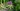# JavaScript 中的 NaN 和 Infinity

## NaN

JavaScript 中的值 `NaN` 用来代表 "not a number"(不是一个数字)。它主要表示转换字符串发生错误：

``Number('xyz'); // NaN``

`NaN` 有一些特殊特性。它的名称是 "not a number"(不是一个数字)，但是却不等于自身：

``NaN !== NaN; // true``

``typeof NaN; // 'number``

### 检测 NaN

`NaN` 是唯一不等于它自身的 JavaScript 值。没有相等的操作，你只能使用全局函数 `isNaN()` 来检测它。

``isNaN(NaN); // true``

`isNaN()` 的一个缺陷是：它首先强制转换参数为数字类型，因此对那些无法转化为数字的字符串使用时将返回 `true`

``````Number('xyz'); // NaN
isNaN('xyz'); // true``````

``````Number({}); // NaN
isNaN({}); // true

Number(['xzy']); // NaN
isNaN(['xzy']); // true``````

``````const obj = {
valueOf: function () {
return NaN;
},
};
Number(obj); // NaN
isNaN(obj); //true``````

``````function myIsNaN(x) {
return x !== x;
}``````

ECMAScript 6 添加了一个新的版本 `Number.isNaN()`，规范的方法比上面的建议更好。它看起来像下面这样：

``````Number.isNaN = function (value) {
return typeof value === 'number' && isNaN(value);
};``````

``````Number.isNaN(NaN); // true
Number.isNaN('xyz'); // false
Number.isNaN({}); // false``````

## Infinity

``````3 / 0; // Infinity
-3 / 0; // -Infinity
0 / 0; // NaN``````

``Infinity - Infinity; // NaN``

``````Infinity + Infinity; // Infinity

5 * Infinity; // Infinity``````